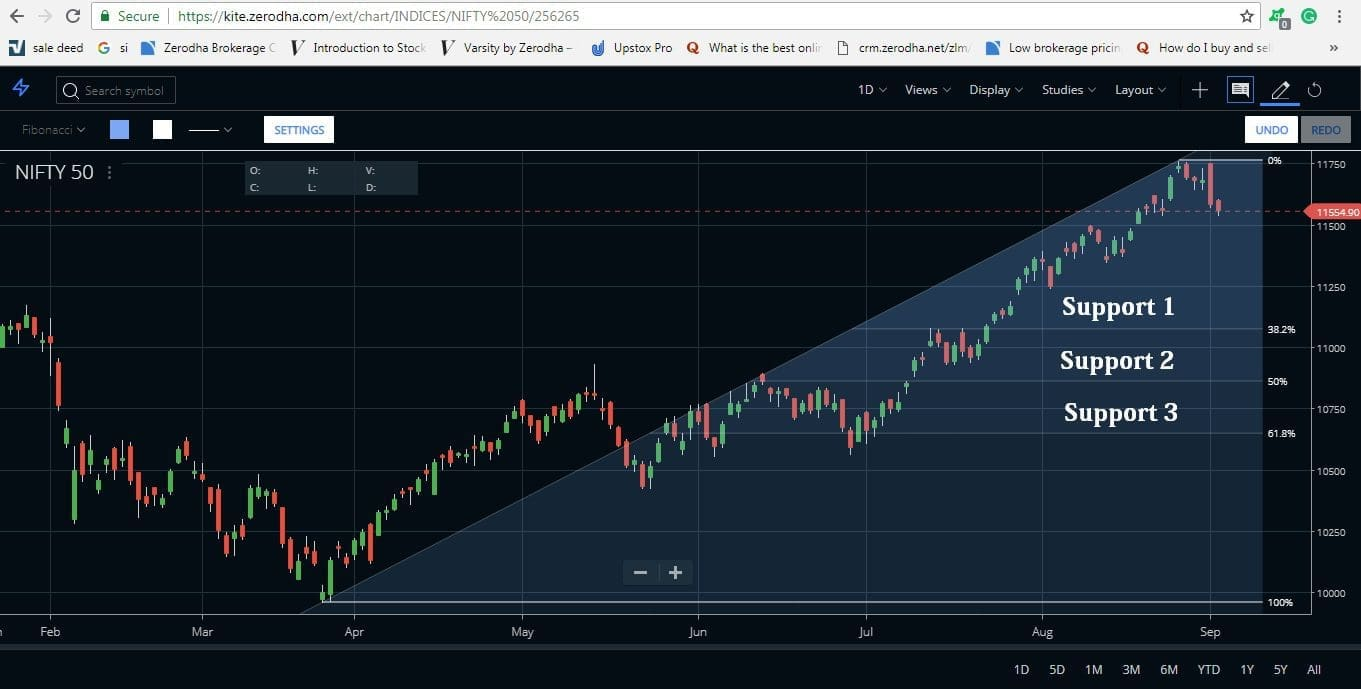Fibonacci Retracement Strategy in the Technical AnalysisPreviously, we have discussed various tools and indicators which come under the technical analysis. Today we come with a completely new tool, term as Fibonacci Retracement. Basically, it works for identifying the support-resistance level in the stock market. Before proceeding to the Fibonacci Retracement, it’s important to know the origin of the particular strategy. The tool is derived from a popular series, Fibonacci Series, developed by Italian mathematician Leonardo Pisano Bogollo, also known as Fibonacci. Without understanding the series, you can’t go further in the Fibonacci Retracement Strategy. Hence, let’s begin with the very basic concept.

History of Fibonacci Retracement Strategy

Leonardo Pisano introduced a series of inter-related numbers which starts from zero. This series includes a sequence of numbers in a specific way that the value of any number in the series is the sum of the previous two numbers.

The Fibonacci series starts like this:

0 , 1, 1, 2, 3, 5, 8, 13, 21, 34,  55, 89, 144, 233, 377…

Observe the above sequence, each number is the sum of its previous two numbers. With the progress of the sequence, a ratio is developed, refers to the golden ratio. The entire sequential ratio is described below:

First, have a look at the sum rule:

For example:

5+8=13
8+13=21
13+21=54
21+34=55

Golden Ratio Calculation:

We can extend the series to infinity. The Golden Fibonacci Ratio comes from the division of any number by its previous number in the series. For example:

377/233 = 1.618
233/144 = 1.618

In order to calculate the consistency of further ratio properties, a number is being divided by its immediate succeeding number and expressed in percentage.

(144/233)x100 = 61.8%
(377/610)x100 = 61.8%

We can find another consistency when a number is divided by a number which is two places higher. After that, it is expressed in percentage.

(13/34)x100= 38.2%
(21/55)x100= 38.2%

When a number is divided by a 3 place higher number, a consistency can be seen:

(13/55)x100 =23.6%.
(21/89)x100 =23.6%.

Application of the Fibonacci Retracement Strategy in the Stock Market

The Fibonacci sequence is quite a popular tool in the stock market. There are certain tools, based on Fibonacci, such as Fibonacci Retracements, Fibonacci Arcs, Fibonacci Fans, Fibonacci Time Extensions. The center point of the article is the Fibonacci Retracements. The first question may arise about the subject is what is meant by the term “retracement”. A retracement refers to a temporary reversal against the prevailing trend. For instance, during an uptrend, a brief period of retracement also known as a dip.

Fibonacci retracements are the simple and widely used Fibonacci tool. Besides identifying the support-resistance level, target and stop loss also an important outcome from it. After applying the retracements tool, some horizontal lines appear along with the indications of the support-resistance level. The calculation is done by the first locating high-low of the chart.

The ratios are 23.6%, 38.2%, 50%, 61.8%…here 50% is not an Fibonacci number. It is used here only because it considers as the potential reversal level.

Example of Fibonacci Retracement StrategyIn order to clear the subject, two sets of images are attached. Observe the first image where the support-resistance level is clearly shown in the ratios of 23.6%, 50%, 38.2%. The tool carries a very simple strategy. Here, at the 23.6% level after getting a resistance, the price will go up and come back to the previous 23.6% level as a support. The retracement is clearly shown here.

For the next example, after applying the Fibonacci line on the NIFTY 50 chart, I have taken a screenshot from Zerodha Kite. Here it is,As you can see in the above chart, as the price recently falls from an up-trend position, if you sell at this moment, you can take 1st support at 38.2%, 2nd support at 50% and 3rd support at the 61.8%. The 50% and 61.8% are considered the most significant points. Here, one thing should be clear to you, a support can works as a resistance level too during an uptrend.

Features of Fibonacci Retracement Strategy

• One of the most simple models among the  Fibonacci tools.
• Identify the support-resistance level.
• Besides support-resistance, the targetstop loss also a significant outcome.
• An entry-exit point can be found easily here.
• Traders can apply this tool in future-options also.

Conclusion

However, this overview regarding Fibonacci Retracement Strategy will guide you to find the proper support-resistance in a stock. For more information, you can check out our another article on the same subject.

Sometimes, the calculation might get tricky to you, you may apply the Fibonacci calculator for the easy calculation method.

This site uses Akismet to reduce spam. Learn how your comment data is processed.